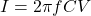## An AC source is connected to a series combination of a light bulb and a variable capacitor. If the capacitance is increased, the bulb’s brig

Question

An AC source is connected to a series combination of a light bulb and a variable capacitor. If the capacitance is increased, the bulb’s brightness A. Increase. B. Decreases C. Does not change.

in progress 0
2 months 2021-07-31T19:36:50+00:00 1 Answers 6 views 0

Increases

Explanation:

Since power P=IV

Then it means when current increases, the power increases hence brightness increases. I represent current, P is power and v is voltage.

Current of capacitor when in series connection is given bywhere I is current across capacitor, f is frequency, C is capacitance and v is voltage across capacitance. From this second formula, it is evident that an increase in capacitance increases the current across the capacitor. Therefore, if current increases, power also increases leading to an increase in brightness